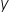Mathematics
Easy

Question

# For a relation, whereis a function of x, and y = 1 when x = 0; which of the following does not represent another possible mapping in the relation?

## x = 4 maps to y = 5    x = 0 maps to y = 0    x = 1 maps to y = 2    x = 3 maps to y = 4Hint:

## The correct answer is: x = 0 maps to y = 0

### Related Questions to study#### With Turito Foundation.#### Get an Expert Advice From Turito.